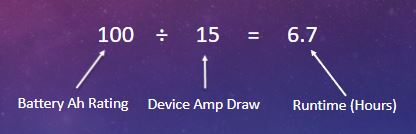# Guide to Buying the Right Size Battery

Purchasing the proper sized battery for your application can be intimidating. Too small, and you find yourself running out of juice too soon. Too big, and you may be spending extra money when you didn’t have to. To understand calculating our perfect battery size, we must first learn a few basic definitions.

## Volt or Voltage (V):

Voltage is the pressure from a power source that is pushing electrons through a loop, enabling them to do work such as power a trolling motor or turn on a light bulb. Batteries are marked with a nominal voltage that is fixed (such as 12, 24, 36, or 48 volts). On the other hand, electronic devices will always operate when receiving a fixed voltage. For example, a 12-volt device needs a battery that supplies 12 volts to operate.

## Current – Ampere (A):

If voltage is the pressure, current is the flow rate. An ampere, or amp, is the international unit used for measuring current. Amp's measure how much electricity “flows” per second. As the rate of current goes up, the measure of amps will also go up. An electronic device usually works on a fixed voltage, but the number of amps it draws can vary. One example is a dimmer light switch, as you slowly raise the dimmer switch you are slowly increasing the amps being sent to the light bulb. The voltage does not change, but the increase in amps will increase the brightness of the light bulb. The same holds true with operating a trolling motor.

Example: I have a 55 lb trolling motor running on speed setting 4. The trolling engine runs on 12V and draws 15A at that speed. If I increase to speed setting 8. The motor still runs on 12V but now pulls 30A. The voltage remains the same but the number of amps increased.

## Power – Watts (W):

Power is volts multiplied by amps, or W = V x A. Power is generally measured in watts (W). This is the amount of energy consumed by a device and therefore an indication of how powerful it is.

Example: I have a 12V trolling motor that draws 30 amps. The power consumption is 12 x 30 = 360W.

It is important to note that power can change on the same device if the amps are increased.

Example: I have a 24V trolling motor that I am running on speed setting 3. The motor runs on 24V and draws 10A. This would give it a power consumption of 240W (24 x 10 = 240). If I increase my speed setting to 5, the motor now draws 15A, but still runs on 24V. The power consumption of the trolling motor is now 360W (24 x 15 = 360).

## Capacity – Amp hours (Ah):

Amp hours, or Ah, is a measurement of battery capacity. The Ah rating of a battery tells us how many amps the battery can deliver in one hour. Higher Ah rating means more runtime. As an example, a 12V lithium battery with a capacity of 100Ah can deliver 100Ah to a 12-volt device for one hour. This also means that the same 100Ah battery could operate a 12V device for 4 hours if the amp draw was 25 amps (100 ÷ 25 = 4). When measuring capacity, we see two values: rated capacity and usable capacity. When shopping for batteries, it is important to understand lithium batteries have a significantly higher proportion of usable capacity than lead acid batteries. For more information about the differences between these two battery types, check out this article.

Example: I run my 55 lb trolling motor at speed setting 4, drawing 15A at 12V. I have a 12-volt battery of 100 Ah. My total run time is now 6.7 hours (100 ÷ 15 = 6.7). When I switch to speed setting 8 the motor draws 30A. My total runtime is now 3.3 hours (100 ÷ 30 = 3.3).## Capacity – Watt-hour (Wh):

Another commonly used terms to measure battery capacity is Watt-hour, or Wh. Wh is calculated by multiplying battery voltage and amp hours. For example, a 12v 54Ah battery would have 648Wh (12 × 54 = 648). A 36v 100Ah battery has 3600Wh (36 × 100 = 3600).

## Battery Size Calculator

Step 1: Determine your battery voltage. Is your application 12V, 24V, or 36V?

Step 2: What is your device’s max intended amp draw?

Step 3: What is your target operating time for this battery at max amp draw?

Use the calculator below to help determine the proper Ah rating you will need from your battery.

Max Amp Draw:
Desired Runtime (hrs):

Battery Ah Rating Required :